Courses

# Nuclear Physics NAT Level – 2

## 10 Questions MCQ Test Topic wise Tests for IIT JAM Physics | Nuclear Physics NAT Level – 2

Description
This mock test of Nuclear Physics NAT Level – 2 for IIT JAM helps you for every IIT JAM entrance exam. This contains 10 Multiple Choice Questions for IIT JAM Nuclear Physics NAT Level – 2 (mcq) to study with solutions a complete question bank. The solved questions answers in this Nuclear Physics NAT Level – 2 quiz give you a good mix of easy questions and tough questions. IIT JAM students definitely take this Nuclear Physics NAT Level – 2 exercise for a better result in the exam. You can find other Nuclear Physics NAT Level – 2 extra questions, long questions & short questions for IIT JAM on EduRev as well by searching above.
*Answer can only contain numeric values
QUESTION: 1

### Graph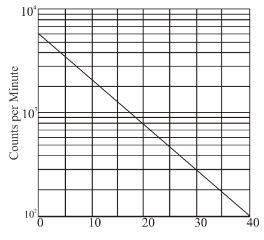A radioactive nucleus decays with the activity shown in the graph above. What is the half life of the nucleus? (in minutes) use log10 2 = 0.3 & log10e = 0.43

Solution:

Count at  t = 0 is 6 × 103
Count at  t  = 30 is 3 × 102
N0 = 6 × 103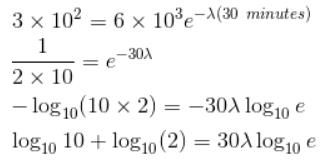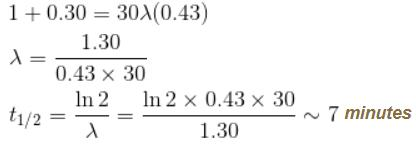*Answer can only contain numeric values
QUESTION: 2

### If the total angular momentum quantum number of a nucleus (A = 200) is J = 1, and if it is due to the rotation of the nucleus as a rigid body, find out the frequency. (in 1012 rad/s)

Solution:

A = 200
mass = 200 × 1.6 × 10–27 kg
J = 1
⇒  Angular momentum =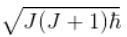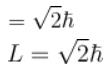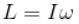(for a rigid body with moment of inertia = I)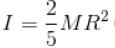(solid sphere since is A is quite high)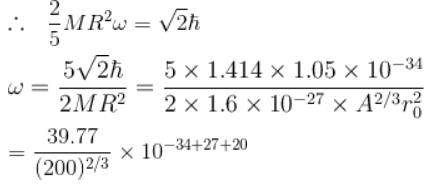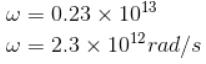*Answer can only contain numeric values
QUESTION: 3

### Plutonium 238Pu94 has an α decay half life of 90 years (2.7 × 109 s). If each of the α particles is emitted with 5.5 MeV energy, then find the average power released (till 50% has decayed) if there are 238 gms of 238Pu (i.e. 6 × 1023 atoms)(in Watts)

Solution:

Solution=

t1/2=2.7x109s

avg power=energy/time

=(No. of atoms decayed x 5.5MeV)/t1/2

=N0x5.5MeV/t1/2

=3x1023x5.5MeV/2.7x109

=(11.6x1023x10+16/109)x10-19

≈116W

*Answer can only contain numeric values
QUESTION: 4

If the energy of the α particle emitted by 231Am is 5.48 MeV, find the distance of closest approach between the α particle and 197Au nucleus (in 10–4 m)

Solution:

K.E, = 5.48 MeV
= 5.48 × 106 × 1.6 × 10-19 J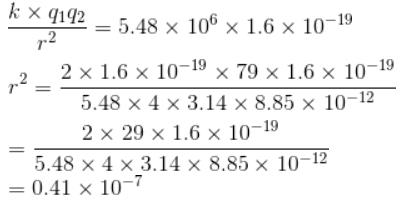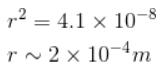*Answer can only contain numeric values
QUESTION: 5

Calculate the typical K.E. expected of an α particle confined within a nucleus if its emitted energy is 10 MeV. (Answer in MeV)

Assume the nuclear potential to be square will of potential V = –10 MeV.

Solution:

If the nuclear potential is a square well
From conservation of energy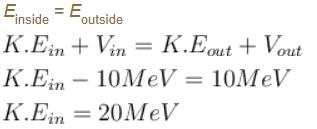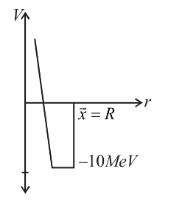*Answer can only contain numeric values
QUESTION: 6

Given that the nucleus density varies with r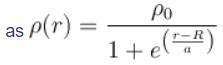where ρ0 = 0.14 nucleon/fm3,  R = 1.07 A1/3 and  a = 0.54  fm.

Find the surface thickness i.e. the distance between which density drops from 0.9ρ0 to 0.1ρ0 (in fm upto two decimal places)

Solution: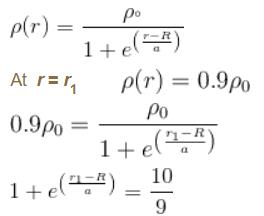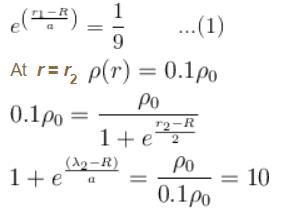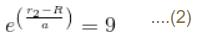Dividing (2) by (1)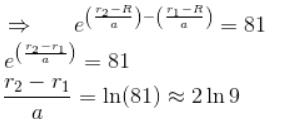Surface thickness = r2 – r1 = a × 4.39
r2 – r1 = 0.54 × 4.39
= 2.37 fm

*Answer can only contain numeric values
QUESTION: 7

The binding energy of a heavy nucleus is about 7 MeV/nucleon, whereas the B.E of a medium weight nucleus is about 8 MeV/nucleon. Therefore the total K.E liberated when a heavy nucleus undergoes symmetric fission is (in MeV).

Solution:

In case of symmetric fission the daughter nuclei have

Ad = A/2 each (daughter nuclei)
K.E. = diff. in B.E.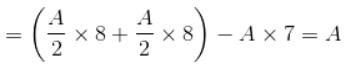For a heavy nucleus A ~ 200
K.E. ~ 200 MeV

*Answer can only contain numeric values
QUESTION: 8

What should be the order of the energy of the electron beam which is to be used to explore the nuclear charge distribution (take the radius to be of the order of 10 fm) and also nucleon charge distribution (r = 0.8 fm)(in GeV)

Solution:

For energy of electron,
λ ~ radius of the nucleus
= 10 fm = 10–14 m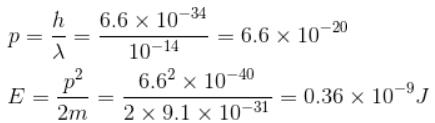= 0.19 × 1010eV ~ 2 × 109eV
~2 GeV

*Answer can only contain numeric values
QUESTION: 9

When α particles are directed onto atoms in a thin metal foil, some make very close collisions with the nuclei of the atom and are scattered at large angles. If an α particle with an initial kinetic energy of 5MeV happens to be scattered through an angle of 180°. Find the distance of closest approach to the scattering nucleus. (in fm)

Solution:

The particle obeys the coulomb's law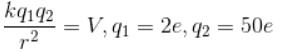(Helium)  (Silver)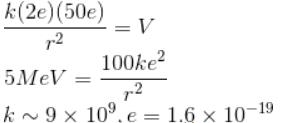In SI units r = 2.9 × 10-14m

*Answer can only contain numeric values
QUESTION: 10

A sample of radioactive nuclei of a certain element can decay only by γ emission and β emission. If half life for γ emission is 24 minutes and that for γ emission  is 36 minutes, the half life for the sample is how much? (in minutes)

Solution:

In such cases, the decay constant is the sum of the different decay constants.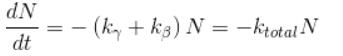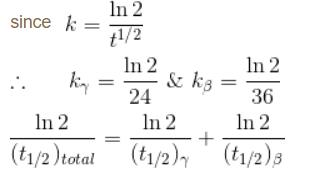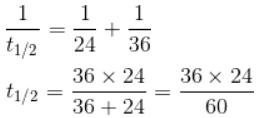t1/2 = 14.4 minutes